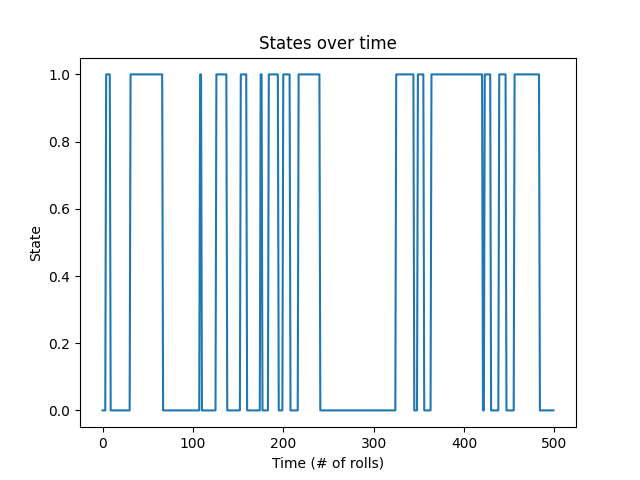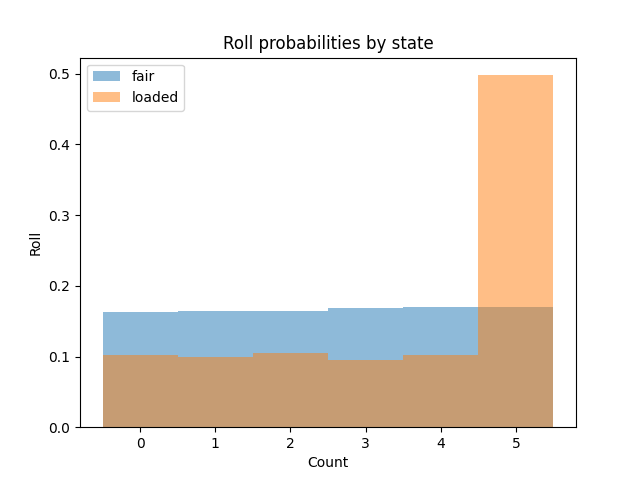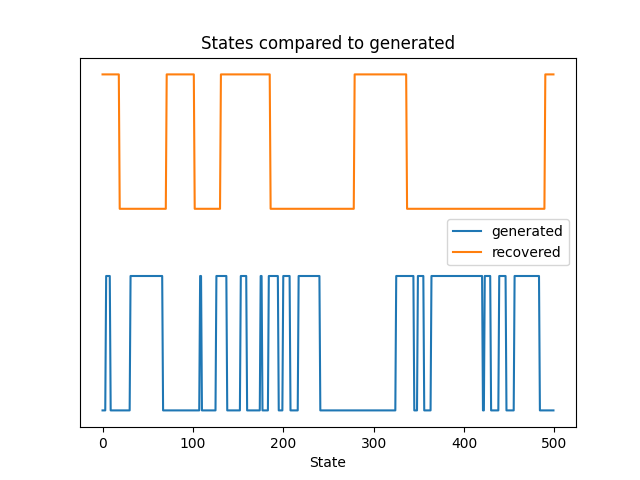# Dishonest Casino Example#

We’ll use the ubiquitous dishonest casino example to demonstrate how to train a Hidden Markov Model (HMM) on somewhat realistic test data (e.g. http://www.mcb111.org/w06/durbin_book.pdf Chapter 3).

In this example, we suspect that a casino is trading out a fair die (singular or dice) for a loaded die. We want to figure out 1) when the loaded die was used (i.e. the most likely path) 2) how often the loaded die is used (i.e. the transition probabilities) and 3) the probabilities for each outcome of a roll for the loaded die (i.e. the emission probabilities).

First, import necessary modules and functions.

```import numpy as np
import matplotlib.pyplot as plt

from hmmlearn import hmm
```

Now, let’s act as the casino and exchange a fair die for a loaded one and generate a series of rolls that someone at the casino would observe.

```# make our generative model with two components, a fair die and a
gen_model = hmm.CategoricalHMM(n_components=2, random_state=99)

# the first state is the fair die so let's start there so no one
# catches on right away
gen_model.startprob_ = np.array([1.0, 0.0])

# now let's say that we sneak the loaded die in:
# here, we have a 95% chance to continue using the fair die and a 5%
# chance to switch to the loaded die
# when we enter the loaded die state, we have a 90% chance of staying
# in that state and a 10% chance of leaving
gen_model.transmat_ = np.array([[0.95, 0.05],
[0.1, 0.9]])

# now let's set the emission means:
# the first state is a fair die with equal probabilities and the
# second is loaded by being biased toward rolling a six
gen_model.emissionprob_ = \
np.array([[1 / 6, 1 / 6, 1 / 6, 1 / 6, 1 / 6, 1 / 6],
[1 / 10, 1 / 10, 1 / 10, 1 / 10, 1 / 10, 1 / 2]])

# simulate the loaded dice rolls
rolls, gen_states = gen_model.sample(30000)

# plot states over time, let's just look at the first rolls for clarity
fig, ax = plt.subplots()
ax.plot(gen_states[:500])
ax.set_title('States over time')
ax.set_xlabel('Time (# of rolls)')
ax.set_ylabel('State')
fig.show()

# plot rolls for the fair and loaded states
fig, ax = plt.subplots()
ax.hist(rolls[gen_states == 0], label='fair', alpha=0.5,
bins=np.arange(7) - 0.5, density=True)
bins=np.arange(7) - 0.5, density=True)
ax.set_title('Roll probabilities by state')
ax.set_xlabel('Count')
ax.set_ylabel('Roll')
ax.legend()
fig.show()
```
••Now, let’s see if we can recover our hidden states, transmission matrix and emission probabilities.

```# split our data into training and validation sets (50/50 split)
X_train = rolls[:rolls.shape // 2]
X_validate = rolls[rolls.shape // 2:]

# check optimal score
gen_score = gen_model.score(X_validate)

best_score = best_model = None
n_fits = 50
np.random.seed(13)
for idx in range(n_fits):
model = hmm.CategoricalHMM(
n_components=2, random_state=idx,
init_params='se')  # don't init transition, set it below
# we need to initialize with random transition matrix probabilities
# because the default is an even likelihood transition
# we know transitions are rare (otherwise the casino would get caught!)
# so let's have an Dirichlet random prior with an alpha value of
# (0.1, 0.9) to enforce our assumption transitions happen roughly 10%
# of the time
model.transmat_ = np.array([np.random.dirichlet([0.9, 0.1]),
np.random.dirichlet([0.1, 0.9])])
model.fit(X_train)
score = model.score(X_validate)
print(f'Model #{idx}\tScore: {score}')
if best_score is None or score > best_score:
best_model = model
best_score = score

print(f'Generated score: {gen_score}\nBest score:      {best_score}')

# use the Viterbi algorithm to predict the most likely sequence of states
# given the model
states = best_model.predict(rolls)

# plot our recovered states compared to generated (aim 1)
fig, ax = plt.subplots()
ax.plot(gen_states[:500], label='generated')
ax.plot(states[:500] + 1.5, label='recovered')
ax.set_yticks([])
ax.set_title('States compared to generated')
ax.set_xlabel('Time (# rolls)')
ax.set_xlabel('State')
ax.legend()
fig.show()
``````Model #0        Score: -26391.366333755435
Model #1        Score: -26395.55036935765
Model #2        Score: -26405.242643523045
Model #3        Score: -26396.290283735074
Model #4        Score: -26395.550365729432
Model #5        Score: -26375.751287784777
Model #6        Score: -26395.484471121286
Model #7        Score: -26300.6743981408
Model #8        Score: -26265.231791844857
Model #9        Score: -26395.55035772545
Model #10       Score: -26317.463400777135
Model #11       Score: -26405.406087293268
Model #12       Score: -26254.5576763989
Model #13       Score: -26395.48203896293
Model #14       Score: -26247.853013151736
Model #15       Score: -26280.573611470674
Model #16       Score: -26236.96934673974
Model #17       Score: -26320.854926121254
Model #18       Score: -26273.89241965078
Model #19       Score: -26404.09350827867
Model #20       Score: -26405.63924373604
Model #21       Score: -26385.763376940657
Model #22       Score: -26395.4853914974
Model #23       Score: -26395.550366095962
Model #24       Score: -26308.4278808233
Model #25       Score: -26395.506893588612
Model #26       Score: -26296.282415126174
Model #27       Score: -26382.710657719595
Model #28       Score: -26393.754915650738
Model #29       Score: -26396.288808293848
Model #30       Score: -26297.051813983053
Model #31       Score: -26282.173855797762
Model #32       Score: -26315.983239191482
Model #33       Score: -26255.80745315831
Model #34       Score: -26309.540391452065
Model #35       Score: -26395.55036583467
Model #36       Score: -26253.060969358412
Model #37       Score: -26395.550513487622
Model #38       Score: -26395.482877957187
Model #39       Score: -26276.49469972745
Model #40       Score: -26395.55036572371
Model #41       Score: -26395.481806494958
Model #42       Score: -26257.756941745938
Model #43       Score: -26395.55037434898
Model #44       Score: -26282.349594637202
Model #45       Score: -26262.652159879224
Model #46       Score: -26344.06860566473
Model #47       Score: -26229.22005031871
Model #48       Score: -26392.68601399797
Model #49       Score: -26336.186925110167
Generated score: -26230.575868403906
Best score:      -26229.22005031871
```

Let’s check our learned transition probabilities and see if they match.

```print(f'Transmission Matrix Generated:\n{gen_model.transmat_.round(3)}\n\n'
f'Transmission Matrix Recovered:\n{best_model.transmat_.round(3)}\n\n')
```
```Transmission Matrix Generated:
[[0.95 0.05]
[0.1  0.9 ]]

Transmission Matrix Recovered:
[[0.922 0.078]
[0.059 0.941]]
```

Finally, let’s see if we can tell how the die is loaded.

```print(f'Emission Matrix Generated:\n{gen_model.emissionprob_.round(3)}\n\n'
f'Emission Matrix Recovered:\n{best_model.emissionprob_.round(3)}\n\n')
```
```Emission Matrix Generated:
[[0.167 0.167 0.167 0.167 0.167 0.167]
[0.1   0.1   0.1   0.1   0.1   0.5  ]]

Emission Matrix Recovered:
[[0.106 0.108 0.114 0.105 0.113 0.454]
[0.168 0.168 0.167 0.171 0.172 0.153]]
```

In this case, we were able to get very good estimates of the transition and emission matrices, but decoding the states was imperfect. That’s because the decoding algorithm is greedy and picks the most likely series of states which isn’t necessarily what happens in real life. Even so, our model could tell us when to watch for the loaded die and we’d have a better chance at catching them red-handed.

Total running time of the script: (0 minutes 4.970 seconds)

Gallery generated by Sphinx-Gallery